Скачать презентацию Lecture Notes Data Structures and Algorithms Chapter 1

5573a0f6c4224676805ee407c4a80aec.ppt

• Количество слайдов: 70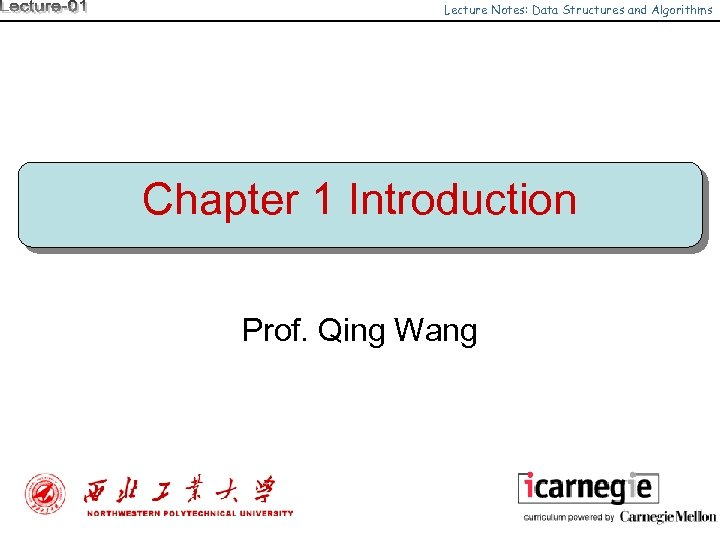Lecture Notes: Data Structures and Algorithms Chapter 1 Introduction Prof. Qing Wang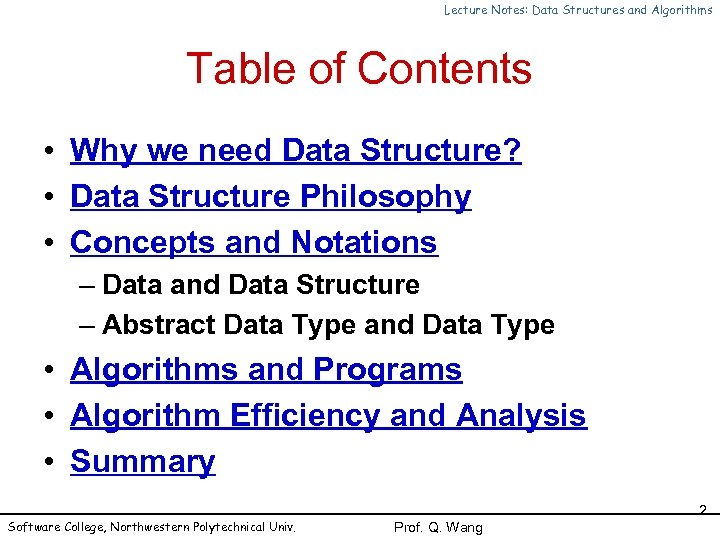Lecture Notes: Data Structures and Algorithms Table of Contents • Why we need Data Structure? • Data Structure Philosophy • Concepts and Notations – Data and Data Structure – Abstract Data Type and Data Type • Algorithms and Programs • Algorithm Efficiency and Analysis • Summary Software College, Northwestern Polytechnical Univ. 2 Prof. Q. Wang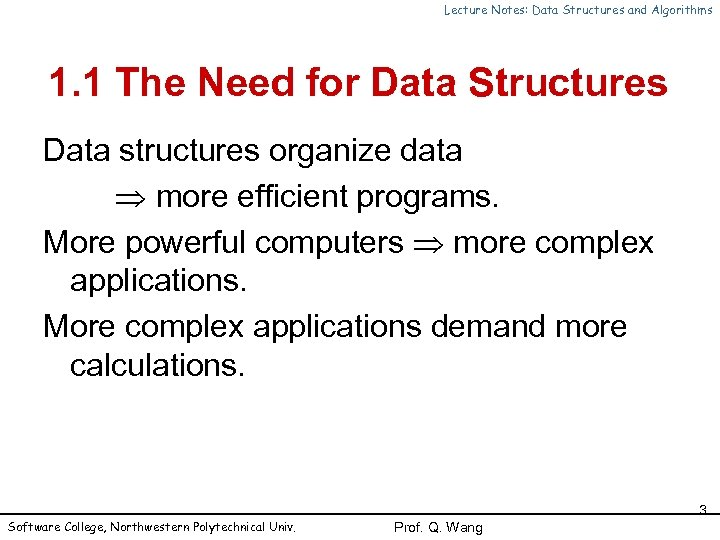Lecture Notes: Data Structures and Algorithms 1. 1 The Need for Data Structures Data structures organize data more efficient programs. More powerful computers more complex applications. More complex applications demand more calculations. Software College, Northwestern Polytechnical Univ. 3 Prof. Q. Wang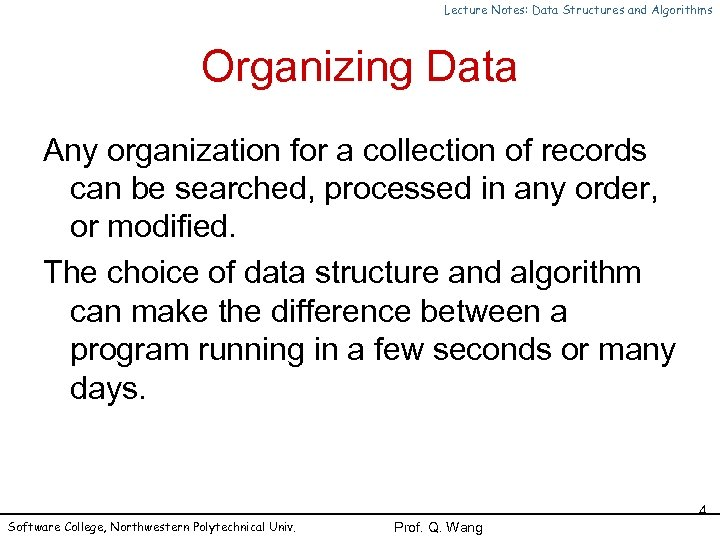Lecture Notes: Data Structures and Algorithms Organizing Data Any organization for a collection of records can be searched, processed in any order, or modified. The choice of data structure and algorithm can make the difference between a program running in a few seconds or many days. Software College, Northwestern Polytechnical Univ. 4 Prof. Q. Wang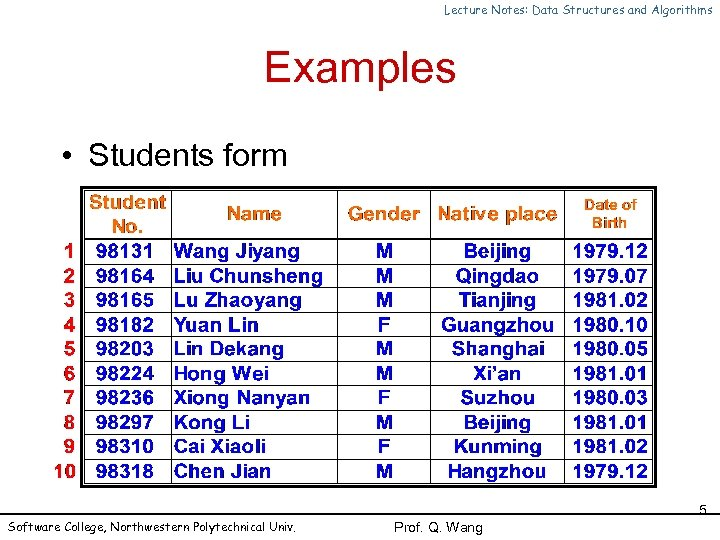Lecture Notes: Data Structures and Algorithms Examples • Students form Software College, Northwestern Polytechnical Univ. 5 Prof. Q. Wang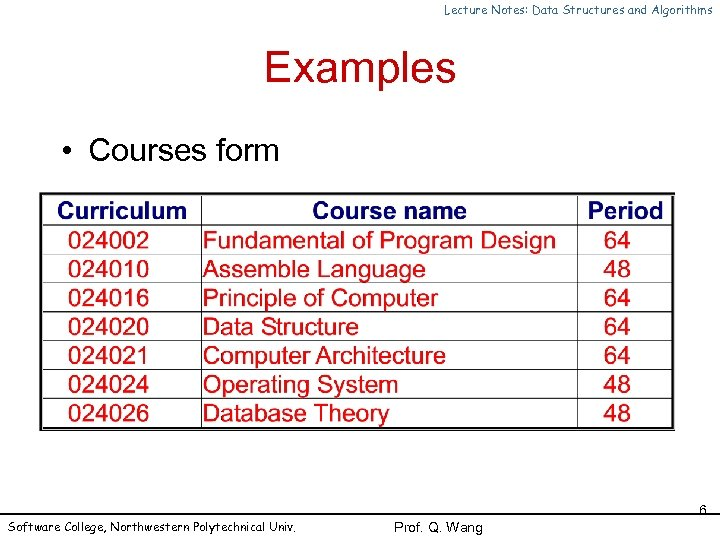Lecture Notes: Data Structures and Algorithms Examples • Courses form Software College, Northwestern Polytechnical Univ. 6 Prof. Q. Wang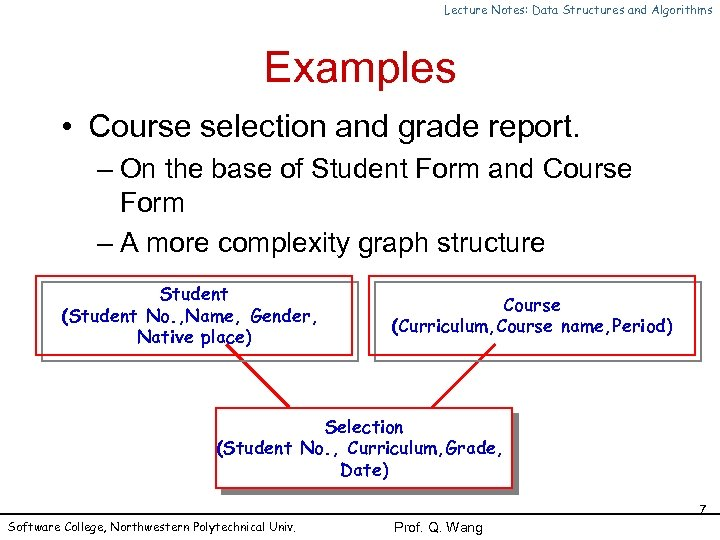Lecture Notes: Data Structures and Algorithms Examples • Course selection and grade report. – On the base of Student Form and Course Form – A more complexity graph structure Student (Student No. , Name, Gender, Native place) Course (Curriculum, Course name, Period) Selection (Student No. , Curriculum, Grade, Date) Software College, Northwestern Polytechnical Univ. 7 Prof. Q. Wang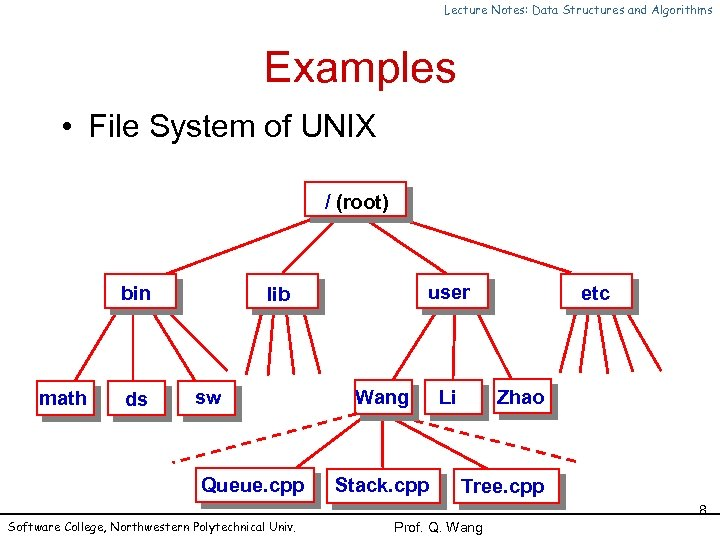Lecture Notes: Data Structures and Algorithms Examples • File System of UNIX / (root) bin math ds user lib sw Queue. cpp Software College, Northwestern Polytechnical Univ. Wang Stack. cpp Li etc Zhao Tree. cpp 8 Prof. Q. Wang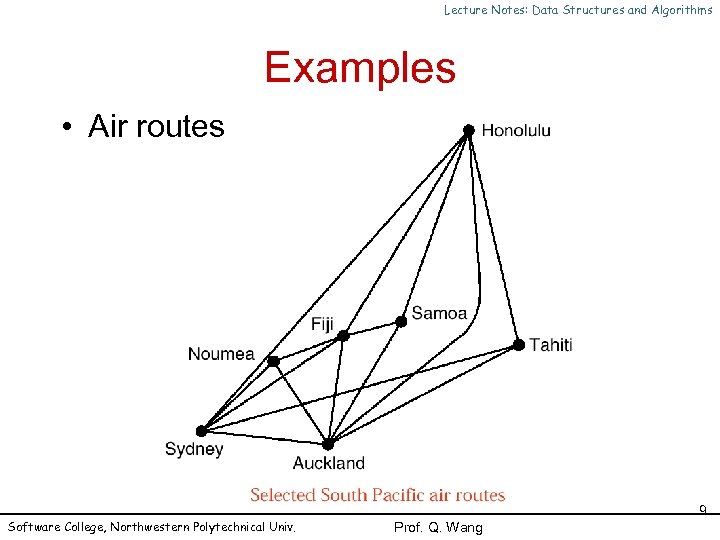Lecture Notes: Data Structures and Algorithms Examples • Air routes Software College, Northwestern Polytechnical Univ. 9 Prof. Q. Wang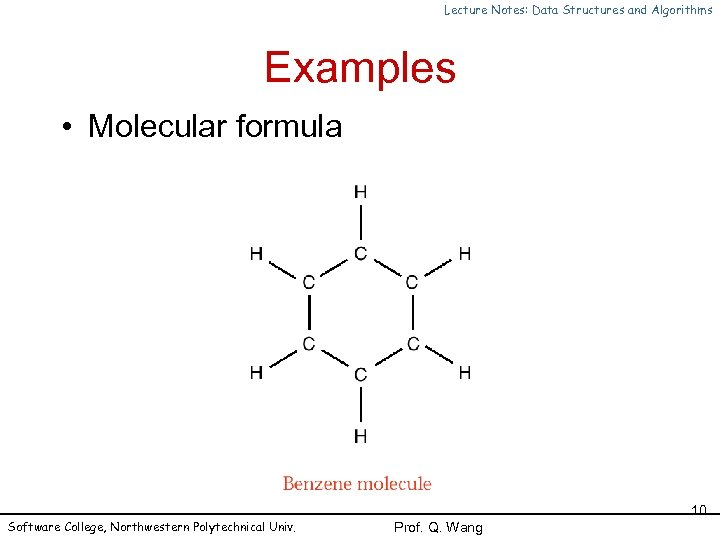Lecture Notes: Data Structures and Algorithms Examples • Molecular formula Software College, Northwestern Polytechnical Univ. 10 Prof. Q. Wang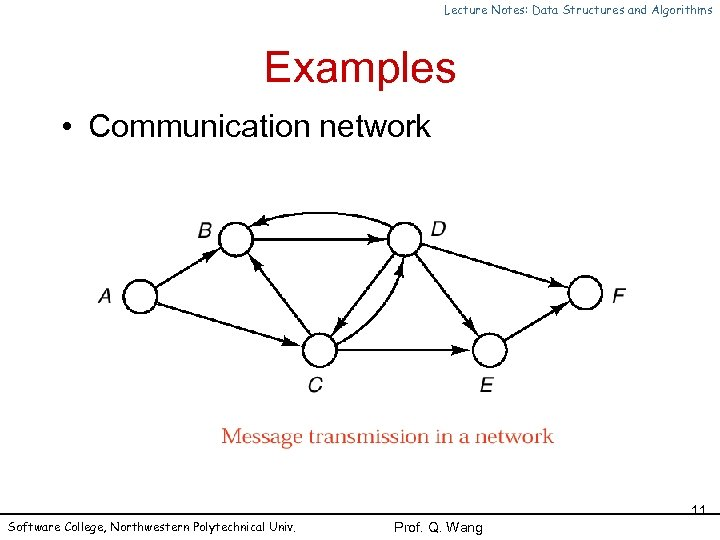Lecture Notes: Data Structures and Algorithms Examples • Communication network Software College, Northwestern Polytechnical Univ. 11 Prof. Q. Wang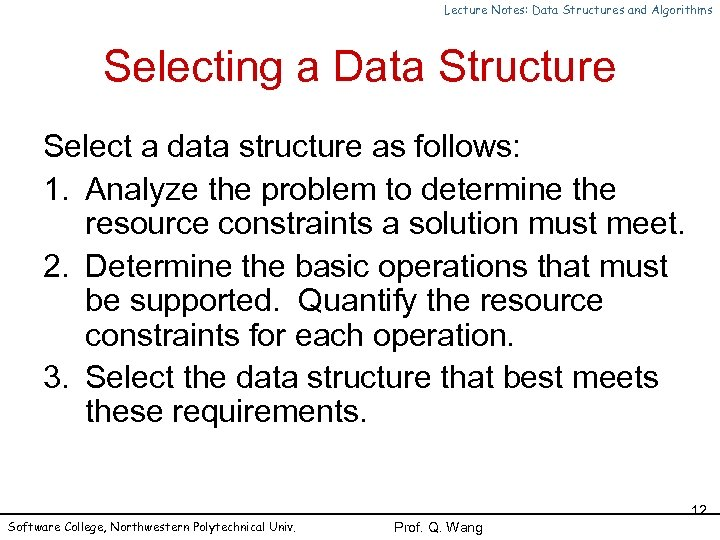Lecture Notes: Data Structures and Algorithms Selecting a Data Structure Select a data structure as follows: 1. Analyze the problem to determine the resource constraints a solution must meet. 2. Determine the basic operations that must be supported. Quantify the resource constraints for each operation. 3. Select the data structure that best meets these requirements. Software College, Northwestern Polytechnical Univ. 12 Prof. Q. Wang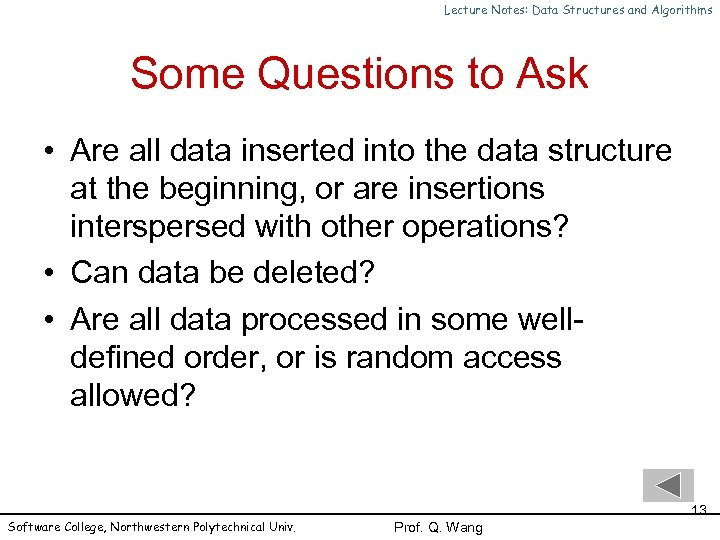Lecture Notes: Data Structures and Algorithms Some Questions to Ask • Are all data inserted into the data structure at the beginning, or are insertions interspersed with other operations? • Can data be deleted? • Are all data processed in some welldefined order, or is random access allowed? Software College, Northwestern Polytechnical Univ. 13 Prof. Q. Wang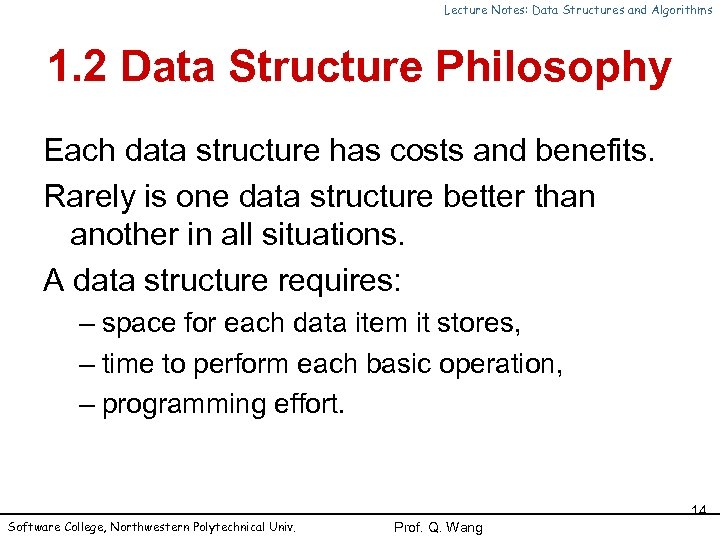Lecture Notes: Data Structures and Algorithms 1. 2 Data Structure Philosophy Each data structure has costs and benefits. Rarely is one data structure better than another in all situations. A data structure requires: – space for each data item it stores, – time to perform each basic operation, – programming effort. Software College, Northwestern Polytechnical Univ. 14 Prof. Q. Wang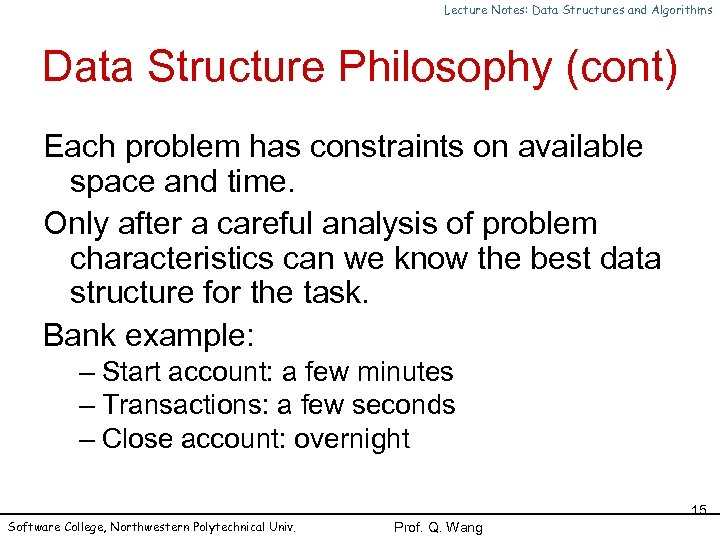Lecture Notes: Data Structures and Algorithms Data Structure Philosophy (cont) Each problem has constraints on available space and time. Only after a careful analysis of problem characteristics can we know the best data structure for the task. Bank example: – Start account: a few minutes – Transactions: a few seconds – Close account: overnight Software College, Northwestern Polytechnical Univ. 15 Prof. Q. Wang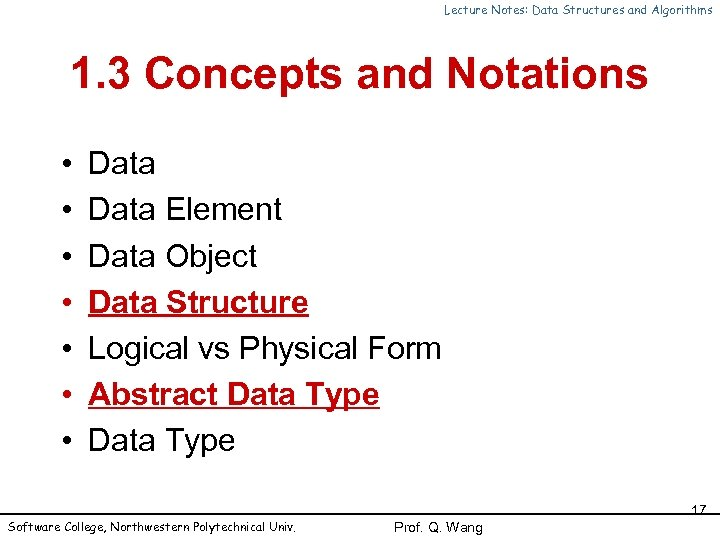Lecture Notes: Data Structures and Algorithms 1. 3 Concepts and Notations • • Data Element Data Object Data Structure Logical vs Physical Form Abstract Data Type Software College, Northwestern Polytechnical Univ. 17 Prof. Q. Wang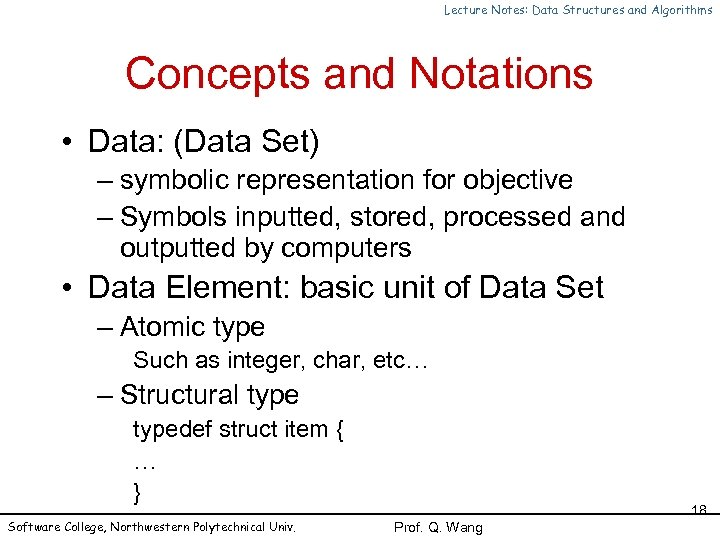Lecture Notes: Data Structures and Algorithms Concepts and Notations • Data: (Data Set) – symbolic representation for objective – Symbols inputted, stored, processed and outputted by computers • Data Element: basic unit of Data Set – Atomic type Such as integer, char, etc… – Structural typedef struct item { … } Software College, Northwestern Polytechnical Univ. 18 Prof. Q. Wang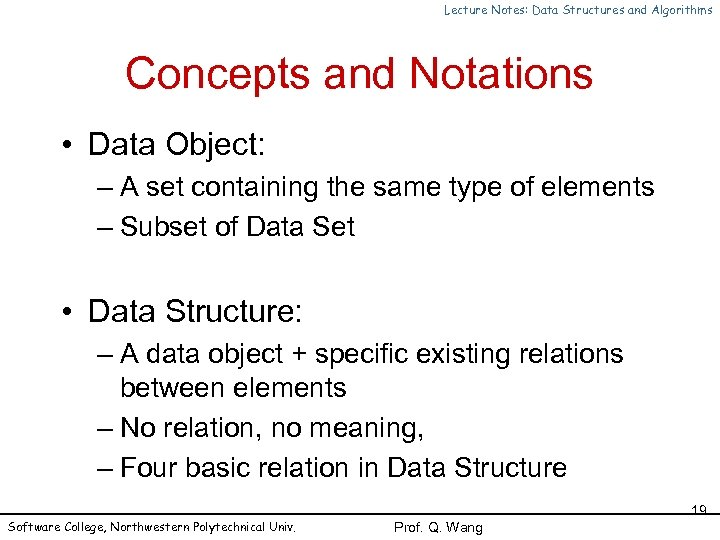Lecture Notes: Data Structures and Algorithms Concepts and Notations • Data Object: – A set containing the same type of elements – Subset of Data Set • Data Structure: – A data object + specific existing relations between elements – No relation, no meaning, – Four basic relation in Data Structure Software College, Northwestern Polytechnical Univ. 19 Prof. Q. Wang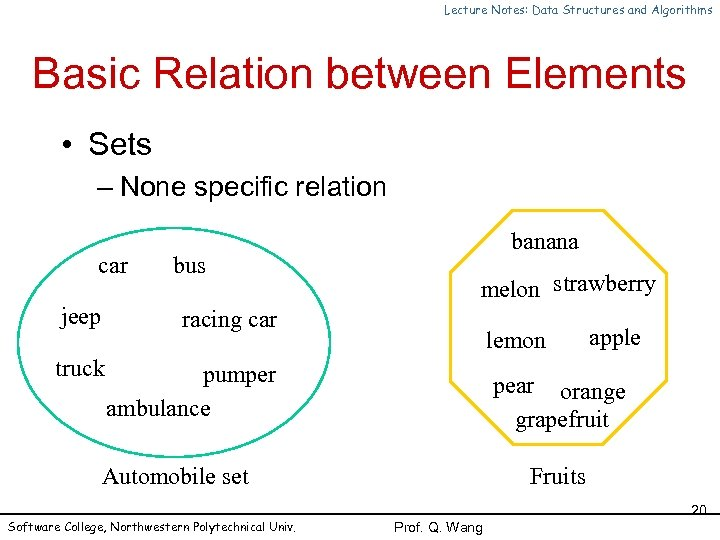Lecture Notes: Data Structures and Algorithms Basic Relation between Elements • Sets – None specific relation car bus jeep melon strawberry racing car truck banana pumper ambulance lemon pear orange grapefruit Automobile set Software College, Northwestern Polytechnical Univ. apple Fruits 20 Prof. Q. Wang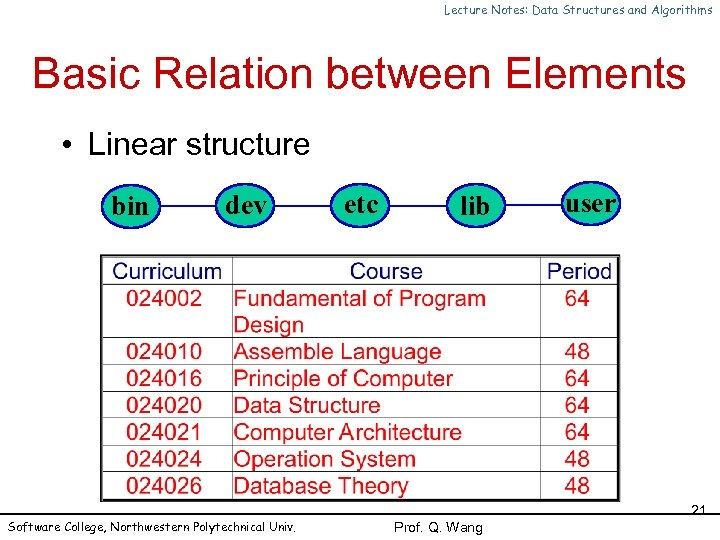Lecture Notes: Data Structures and Algorithms Basic Relation between Elements • Linear structure bin dev Software College, Northwestern Polytechnical Univ. etc lib user 21 Prof. Q. Wang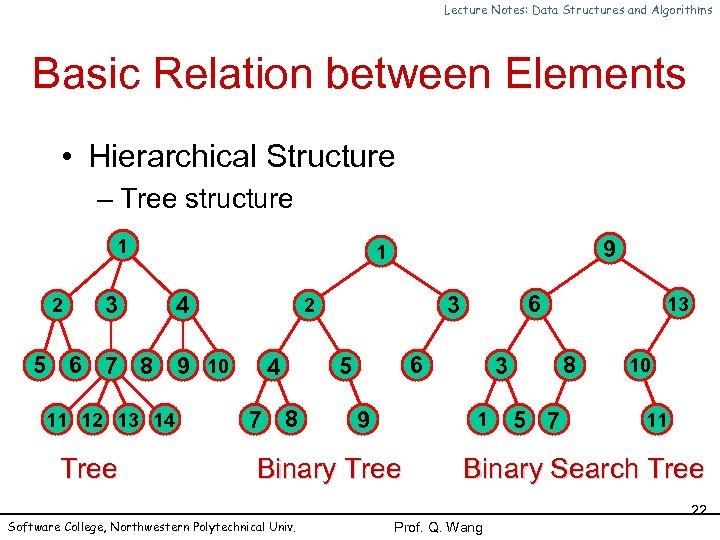Lecture Notes: Data Structures and Algorithms Basic Relation between Elements • Hierarchical Structure – Tree structure 1 2 3 4 5 6 7 8 9 10 11 12 13 14 Tree 9 1 4 7 8 6 5 9 13 8 3 1 Binary Tree Software College, Northwestern Polytechnical Univ. 6 3 2 5 7 10 11 Binary Search Tree 22 Prof. Q. Wang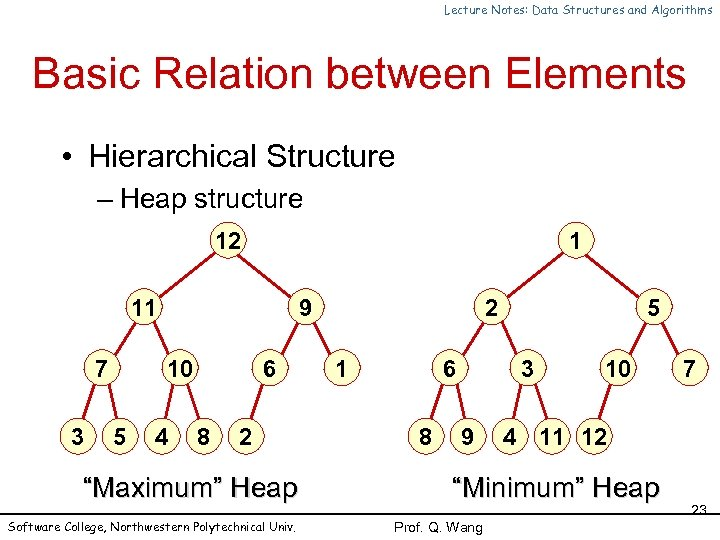Lecture Notes: Data Structures and Algorithms Basic Relation between Elements • Hierarchical Structure – Heap structure 12 1 11 7 3 9 10 5 4 6 8 2 “Maximum” Heap Software College, Northwestern Polytechnical Univ. 2 1 6 8 5 3 9 10 4 11 12 “Minimum” Heap Prof. Q. Wang 7 23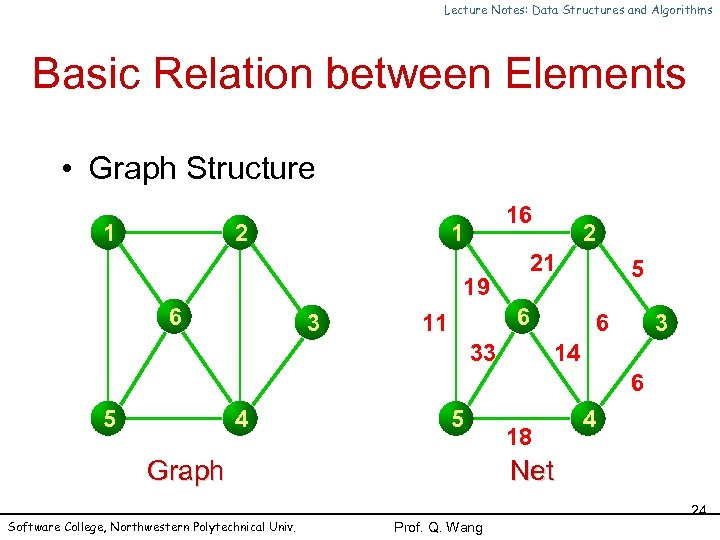Lecture Notes: Data Structures and Algorithms Basic Relation between Elements • Graph Structure 1 2 16 1 19 6 3 2 21 6 11 33 5 6 3 14 6 5 4 5 Graph Software College, Northwestern Polytechnical Univ. 18 4 Net 24 Prof. Q. Wang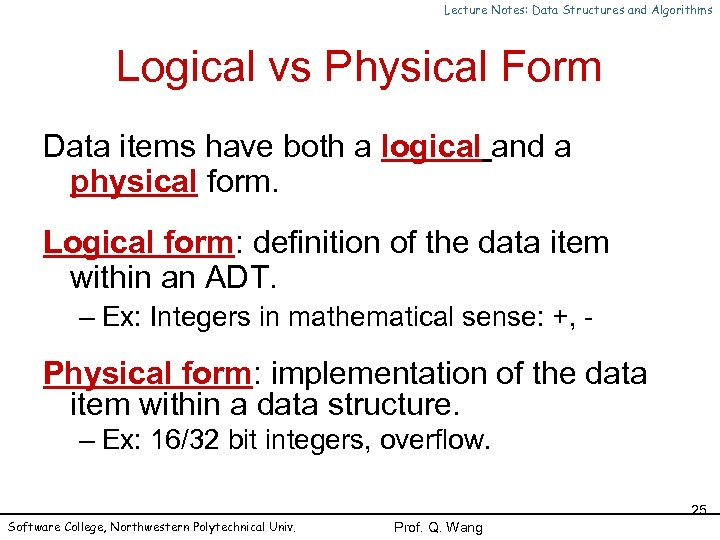Lecture Notes: Data Structures and Algorithms Logical vs Physical Form Data items have both a logical and a physical form. Logical form: definition of the data item within an ADT. – Ex: Integers in mathematical sense: +, - Physical form: implementation of the data item within a data structure. – Ex: 16/32 bit integers, overflow. Software College, Northwestern Polytechnical Univ. 25 Prof. Q. Wang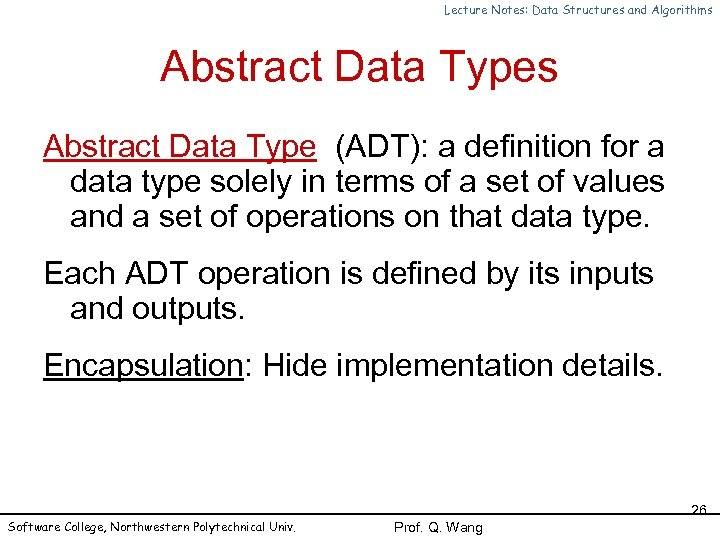Lecture Notes: Data Structures and Algorithms Abstract Data Type (ADT): a definition for a data type solely in terms of a set of values and a set of operations on that data type. Each ADT operation is defined by its inputs and outputs. Encapsulation: Hide implementation details. Software College, Northwestern Polytechnical Univ. 26 Prof. Q. Wang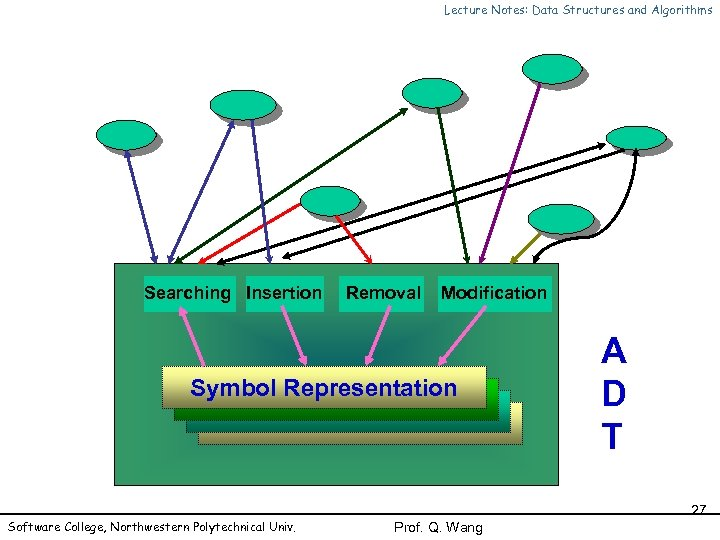Lecture Notes: Data Structures and Algorithms Searching Insertion Removal Modification Symbol Representation Software College, Northwestern Polytechnical Univ. A D T 27 Prof. Q. Wang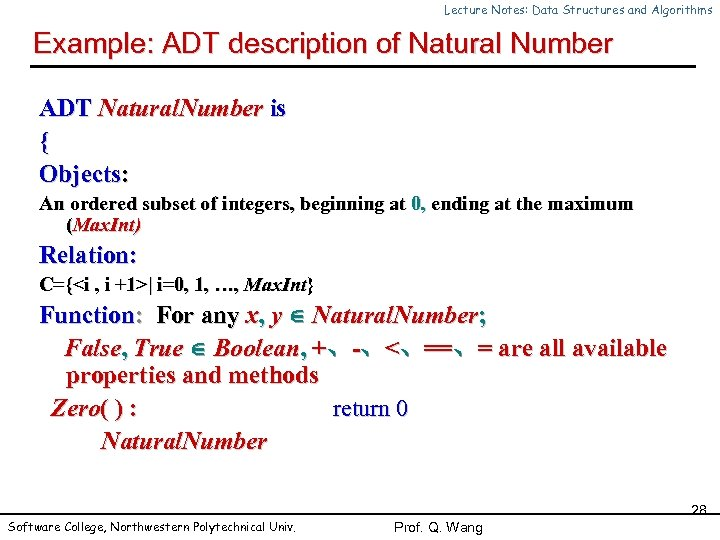Lecture Notes: Data Structures and Algorithms Example: ADT description of Natural Number ADT Natural. Number is { Objects: An ordered subset of integers, beginning at 0, ending at the maximum (Max. Int) Relation: C={| i=0, 1, …, Max. Int} Function: For any x, y Natural. Number; False, True Boolean, +、-、<、==、= are all available properties and methods Zero( ) : return 0 Natural. Number Software College, Northwestern Polytechnical Univ. 28 Prof. Q. Wang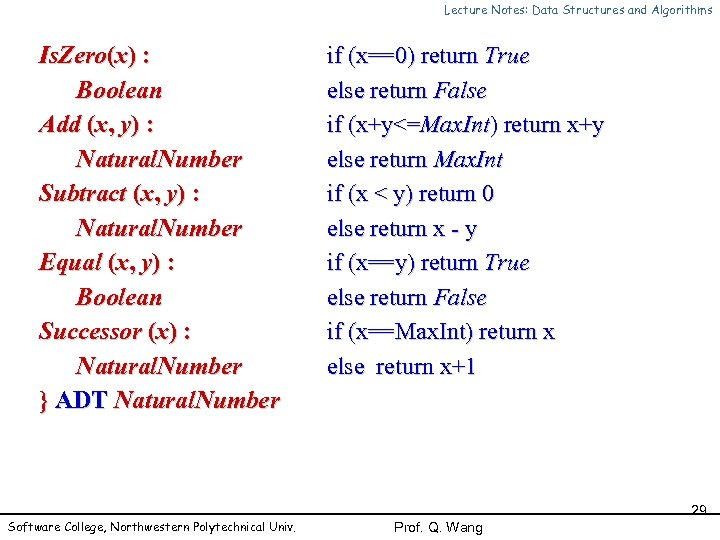Lecture Notes: Data Structures and Algorithms Is. Zero(x) : Boolean Add (x, y) : Natural. Number Subtract (x, y) : Natural. Number Equal (x, y) : Boolean Successor (x) : Natural. Number } ADT Natural. Number Software College, Northwestern Polytechnical Univ. if (x==0) return True else return False if (x+y<=Max. Int) return x+y else return Max. Int if (x < y) return 0 else return x - y if (x==y) return True else return False if (x==Max. Int) return x else return x+1 29 Prof. Q. Wang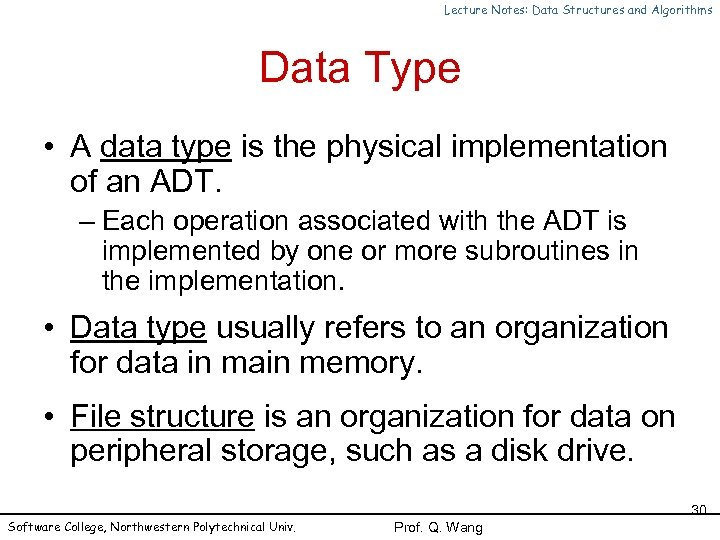Lecture Notes: Data Structures and Algorithms Data Type • A data type is the physical implementation of an ADT. – Each operation associated with the ADT is implemented by one or more subroutines in the implementation. • Data type usually refers to an organization for data in main memory. • File structure is an organization for data on peripheral storage, such as a disk drive. Software College, Northwestern Polytechnical Univ. 30 Prof. Q. Wang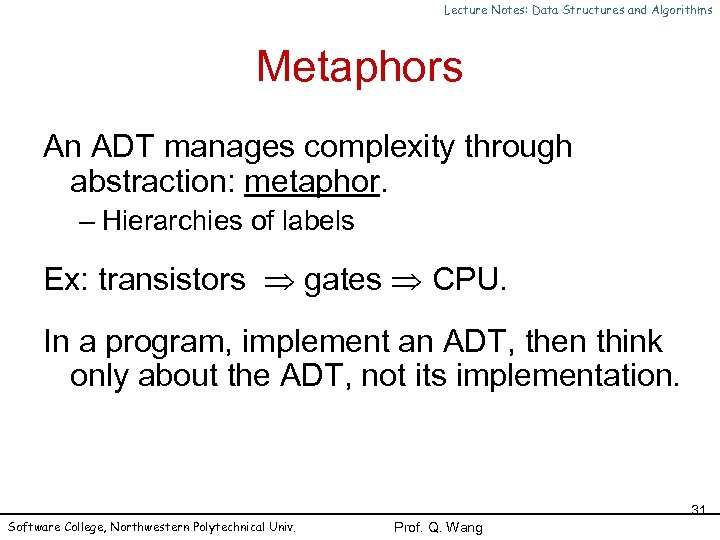Lecture Notes: Data Structures and Algorithms Metaphors An ADT manages complexity through abstraction: metaphor. – Hierarchies of labels Ex: transistors gates CPU. In a program, implement an ADT, then think only about the ADT, not its implementation. Software College, Northwestern Polytechnical Univ. 31 Prof. Q. Wang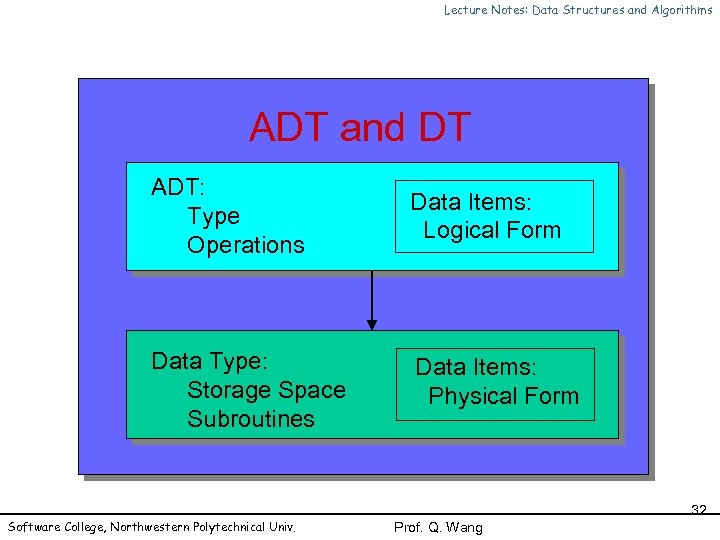Lecture Notes: Data Structures and Algorithms ADT and DT ADT: Type Operations Data Items: Logical Form Data Type: Storage Space Subroutines Data Items: Physical Form Software College, Northwestern Polytechnical Univ. 32 Prof. Q. Wang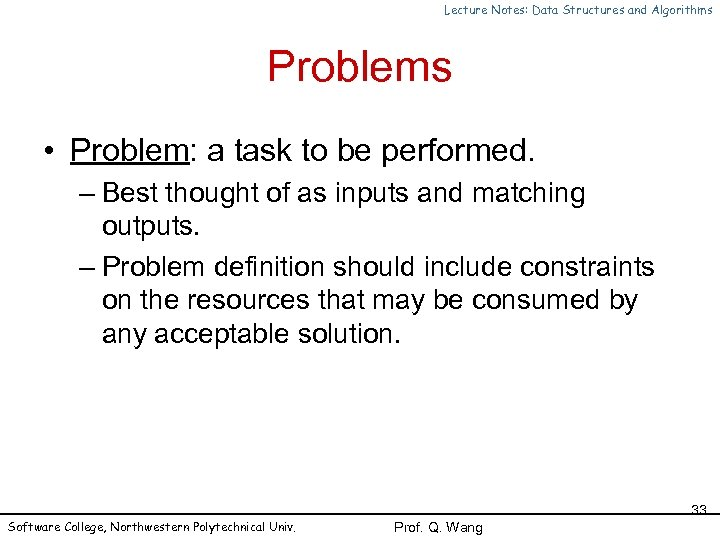Lecture Notes: Data Structures and Algorithms Problems • Problem: a task to be performed. – Best thought of as inputs and matching outputs. – Problem definition should include constraints on the resources that may be consumed by any acceptable solution. Software College, Northwestern Polytechnical Univ. 33 Prof. Q. Wang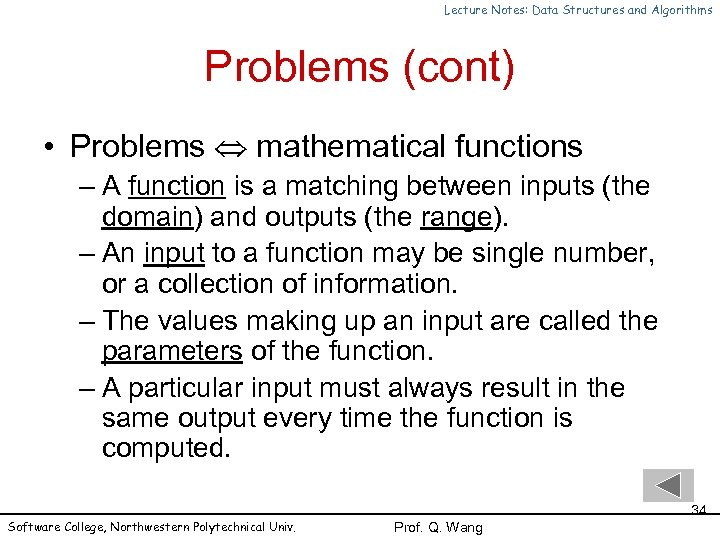Lecture Notes: Data Structures and Algorithms Problems (cont) • Problems mathematical functions – A function is a matching between inputs (the domain) and outputs (the range). – An input to a function may be single number, or a collection of information. – The values making up an input are called the parameters of the function. – A particular input must always result in the same output every time the function is computed. Software College, Northwestern Polytechnical Univ. 34 Prof. Q. Wang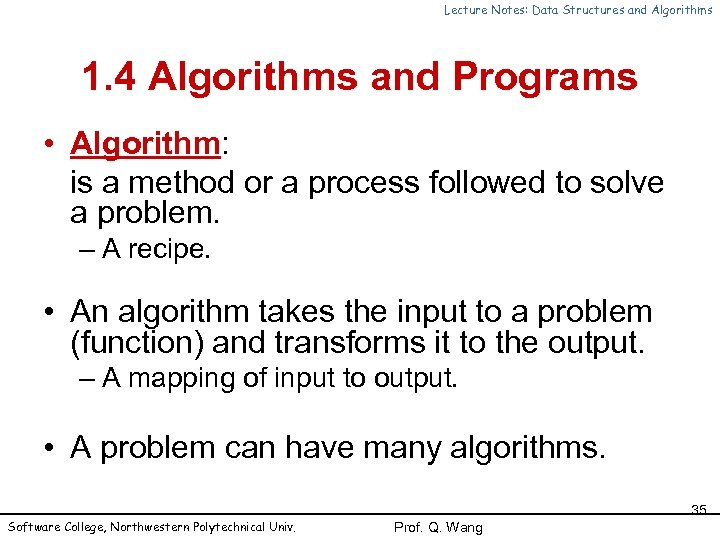Lecture Notes: Data Structures and Algorithms 1. 4 Algorithms and Programs • Algorithm: is a method or a process followed to solve a problem. – A recipe. • An algorithm takes the input to a problem (function) and transforms it to the output. – A mapping of input to output. • A problem can have many algorithms. Software College, Northwestern Polytechnical Univ. 35 Prof. Q. Wang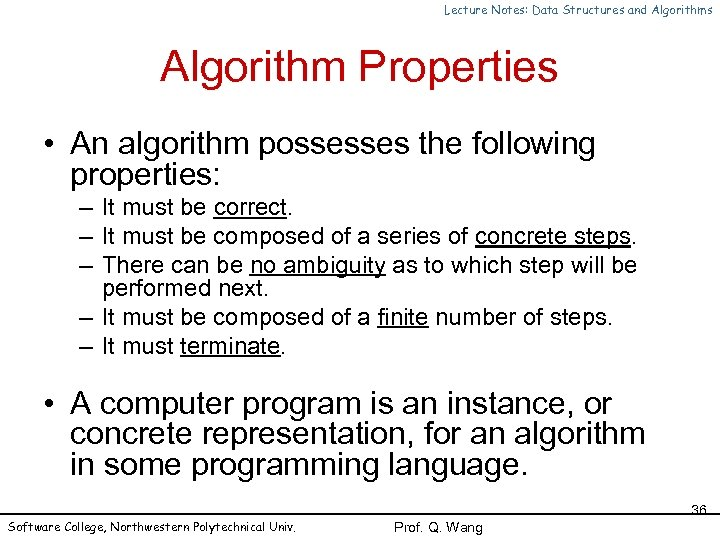Lecture Notes: Data Structures and Algorithms Algorithm Properties • An algorithm possesses the following properties: – It must be correct. – It must be composed of a series of concrete steps. – There can be no ambiguity as to which step will be performed next. – It must be composed of a finite number of steps. – It must terminate. • A computer program is an instance, or concrete representation, for an algorithm in some programming language. Software College, Northwestern Polytechnical Univ. 36 Prof. Q. Wang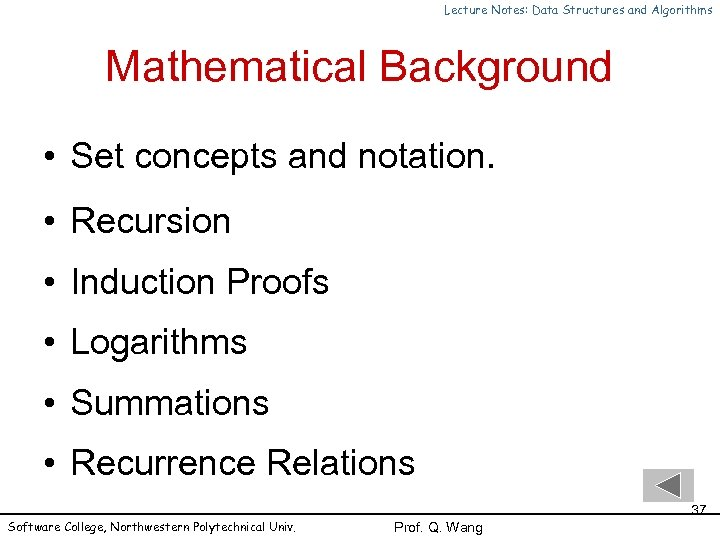Lecture Notes: Data Structures and Algorithms Mathematical Background • Set concepts and notation. • Recursion • Induction Proofs • Logarithms • Summations • Recurrence Relations Software College, Northwestern Polytechnical Univ. 37 Prof. Q. Wang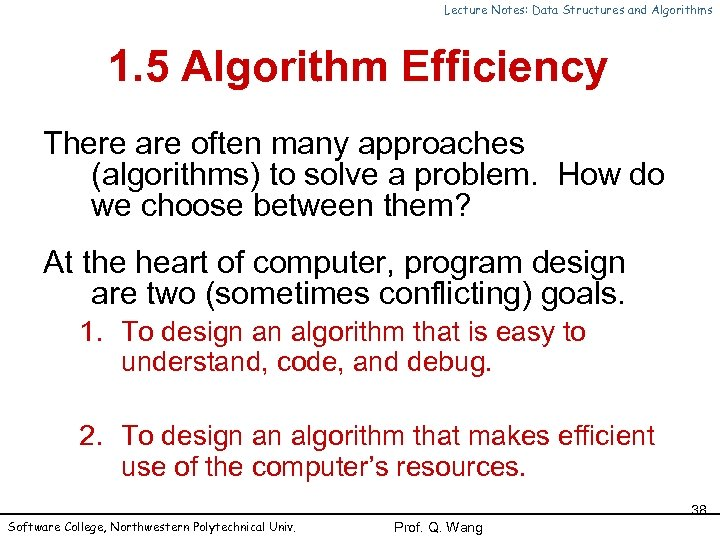Lecture Notes: Data Structures and Algorithms 1. 5 Algorithm Efficiency There are often many approaches (algorithms) to solve a problem. How do we choose between them? At the heart of computer, program design are two (sometimes conflicting) goals. 1. To design an algorithm that is easy to understand, code, and debug. 2. To design an algorithm that makes efficient use of the computer’s resources. Software College, Northwestern Polytechnical Univ. 38 Prof. Q. WangLecture Notes: Data Structures and Algorithms Algorithm Efficiency (cont) Goal (1) is the concern of Software Engineering. Goal (2) is the concern of data structures and algorithm analysis. When goal (2) is important, how do we measure an algorithm’s cost? 1. To design an algorithm that is easy to understand, code, and debug. 2. To design an algorithm that makes efficient use of the computer’s resources. Software College, Northwestern Polytechnical Univ. 39 Prof. Q. Wang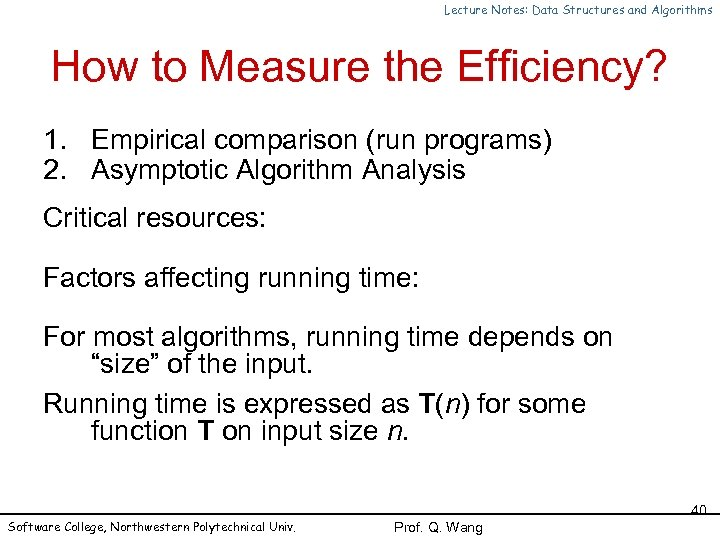Lecture Notes: Data Structures and Algorithms How to Measure the Efficiency? 1. Empirical comparison (run programs) 2. Asymptotic Algorithm Analysis Critical resources: Factors affecting running time: For most algorithms, running time depends on “size” of the input. Running time is expressed as T(n) for some function T on input size n. Software College, Northwestern Polytechnical Univ. 40 Prof. Q. Wang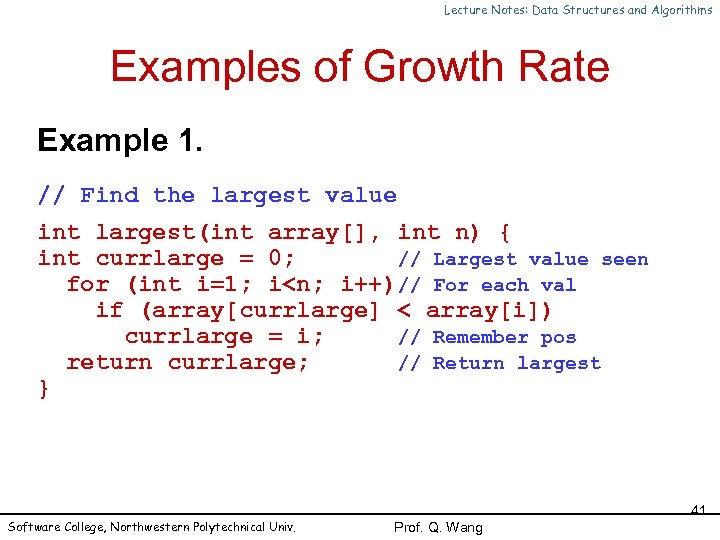Lecture Notes: Data Structures and Algorithms Examples of Growth Rate Example 1. // Find the largest value int largest(int array[], int n) { int currlarge = 0; // Largest value seen for (int i=1; i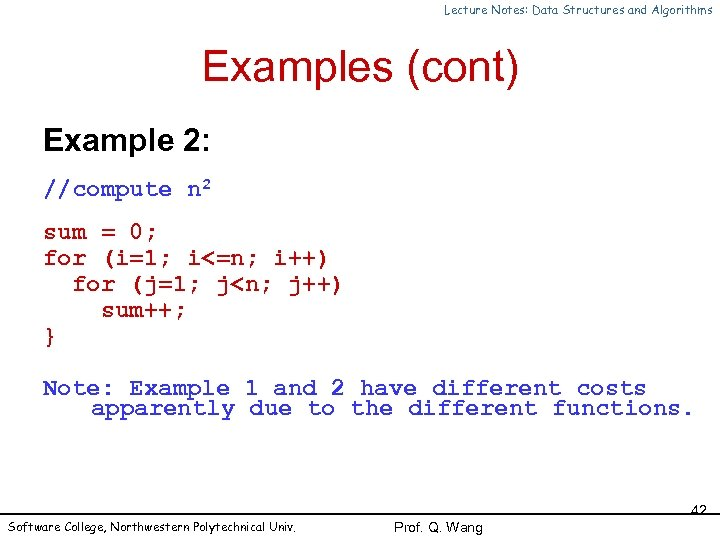Lecture Notes: Data Structures and Algorithms Examples (cont) Example 2: //compute n 2 sum = 0; for (i=1; i<=n; i++) for (j=1; j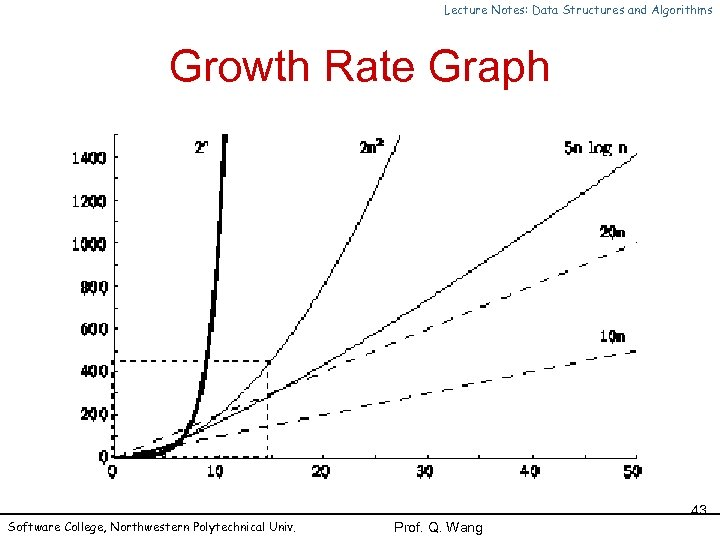Lecture Notes: Data Structures and Algorithms Growth Rate Graph Software College, Northwestern Polytechnical Univ. 43 Prof. Q. Wang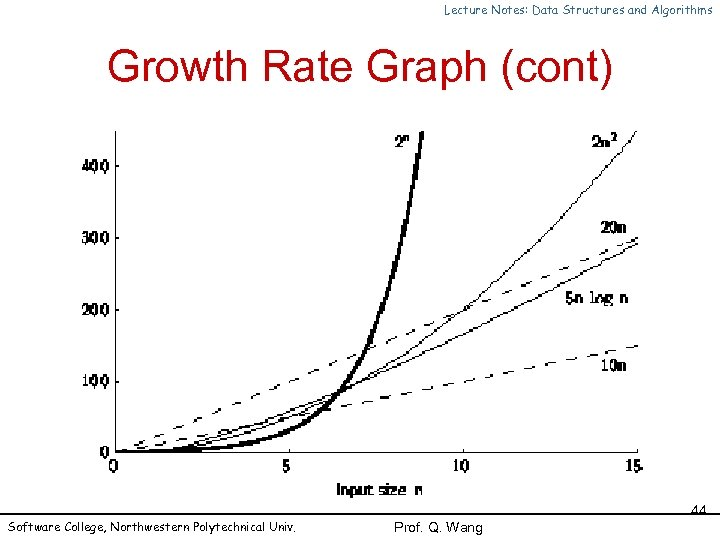Lecture Notes: Data Structures and Algorithms Growth Rate Graph (cont) Software College, Northwestern Polytechnical Univ. 44 Prof. Q. Wang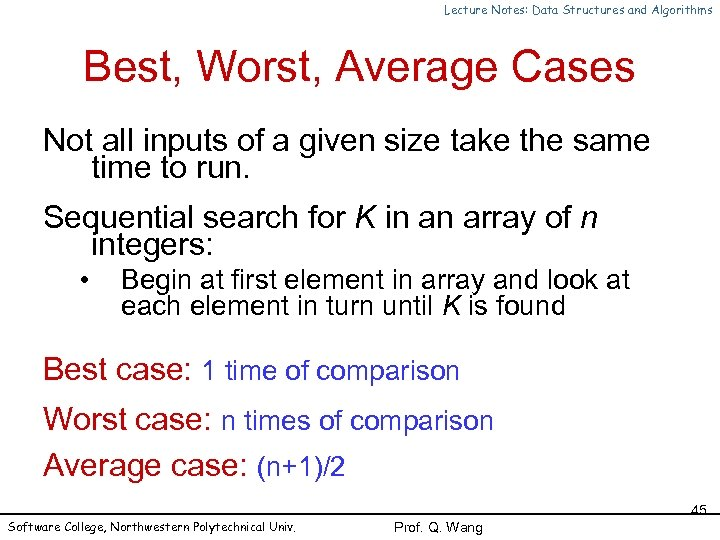Lecture Notes: Data Structures and Algorithms Best, Worst, Average Cases Not all inputs of a given size take the same time to run. Sequential search for K in an array of n integers: • Begin at first element in array and look at each element in turn until K is found Best case: 1 time of comparison Worst case: n times of comparison Average case: (n+1)/2 Software College, Northwestern Polytechnical Univ. 45 Prof. Q. Wang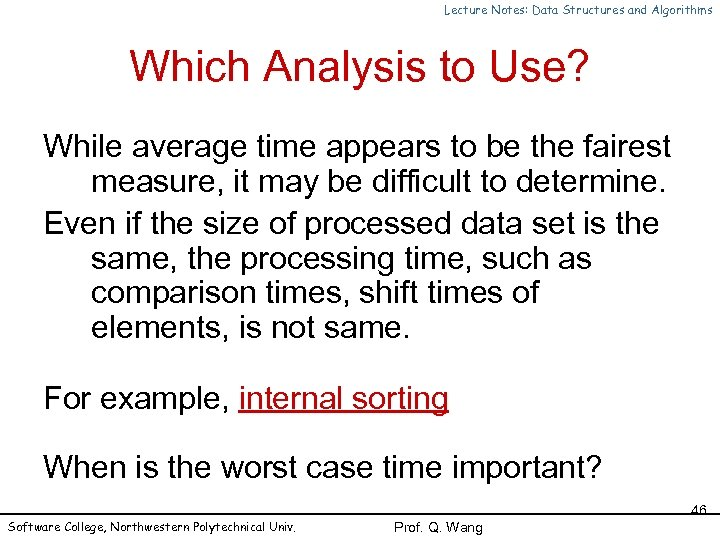Lecture Notes: Data Structures and Algorithms Which Analysis to Use? While average time appears to be the fairest measure, it may be difficult to determine. Even if the size of processed data set is the same, the processing time, such as comparison times, shift times of elements, is not same. For example, internal sorting When is the worst case time important? Software College, Northwestern Polytechnical Univ. 46 Prof. Q. Wang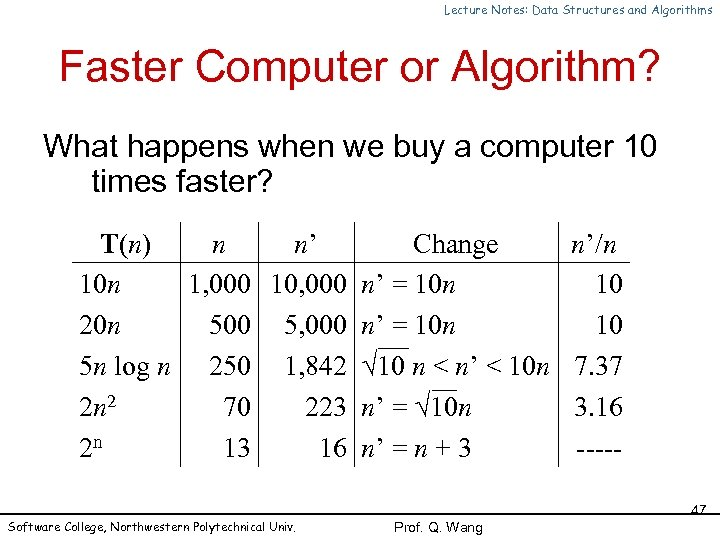Lecture Notes: Data Structures and Algorithms Faster Computer or Algorithm? What happens when we buy a computer 10 times faster? T(n) n n’ Change 10 n 1, 000 10, 000 n’ = 10 n 20 n 500 5, 000 n’ = 10 n 5 n log n 250 1, 842 10 n < n’ < 10 n 2 n 2 70 223 n’ = 10 n 2 n 13 16 n’ = n + 3 Software College, Northwestern Polytechnical Univ. n’/n 10 10 7. 37 3. 16 ----47 Prof. Q. Wang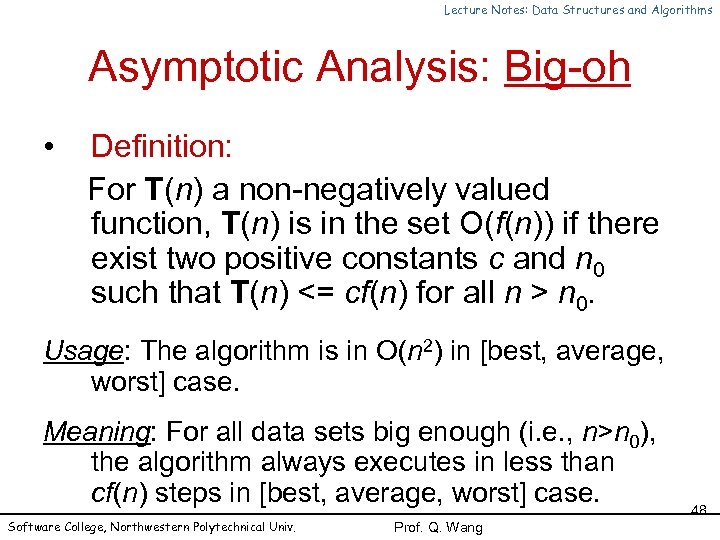Lecture Notes: Data Structures and Algorithms Asymptotic Analysis: Big-oh • Definition: For T(n) a non-negatively valued function, T(n) is in the set O(f(n)) if there exist two positive constants c and n 0 such that T(n) <= cf(n) for all n > n 0. Usage: The algorithm is in O(n 2) in [best, average, worst] case. Meaning: For all data sets big enough (i. e. , n>n 0), the algorithm always executes in less than cf(n) steps in [best, average, worst] case. Software College, Northwestern Polytechnical Univ. Prof. Q. Wang 48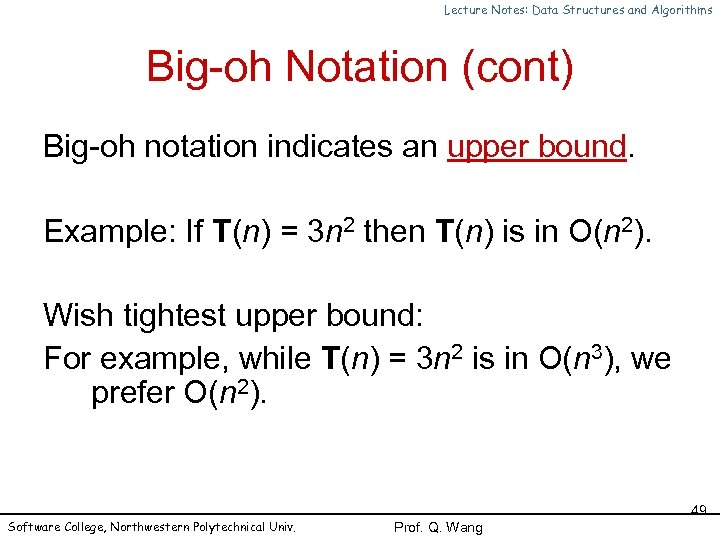Lecture Notes: Data Structures and Algorithms Big-oh Notation (cont) Big-oh notation indicates an upper bound. Example: If T(n) = 3 n 2 then T(n) is in O(n 2). Wish tightest upper bound: For example, while T(n) = 3 n 2 is in O(n 3), we prefer O(n 2). Software College, Northwestern Polytechnical Univ. 49 Prof. Q. Wang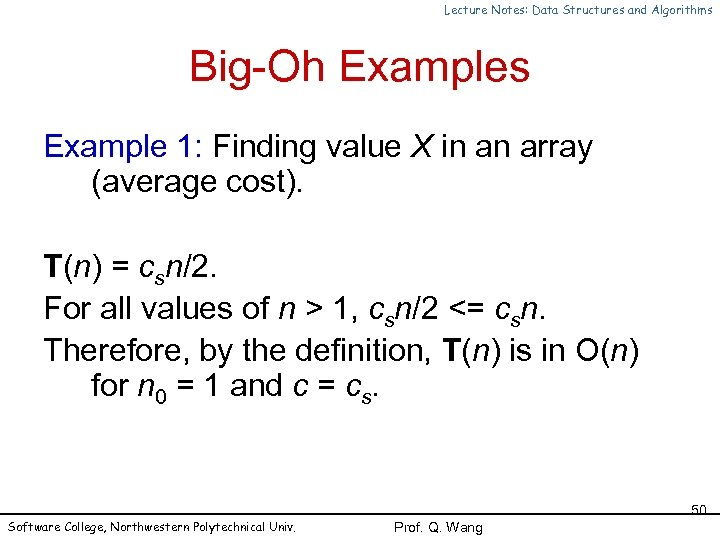Lecture Notes: Data Structures and Algorithms Big-Oh Examples Example 1: Finding value X in an array (average cost). T(n) = csn/2. For all values of n > 1, csn/2 <= csn. Therefore, by the definition, T(n) is in O(n) for n 0 = 1 and c = cs. Software College, Northwestern Polytechnical Univ. 50 Prof. Q. Wang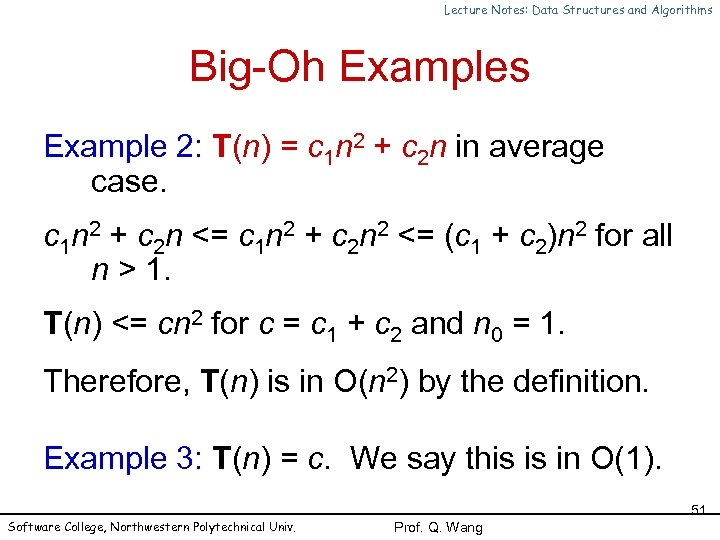Lecture Notes: Data Structures and Algorithms Big-Oh Examples Example 2: T(n) = c 1 n 2 + c 2 n in average case. c 1 n 2 + c 2 n <= c 1 n 2 + c 2 n 2 <= (c 1 + c 2)n 2 for all n > 1. T(n) <= cn 2 for c = c 1 + c 2 and n 0 = 1. Therefore, T(n) is in O(n 2) by the definition. Example 3: T(n) = c. We say this is in O(1). Software College, Northwestern Polytechnical Univ. 51 Prof. Q. Wang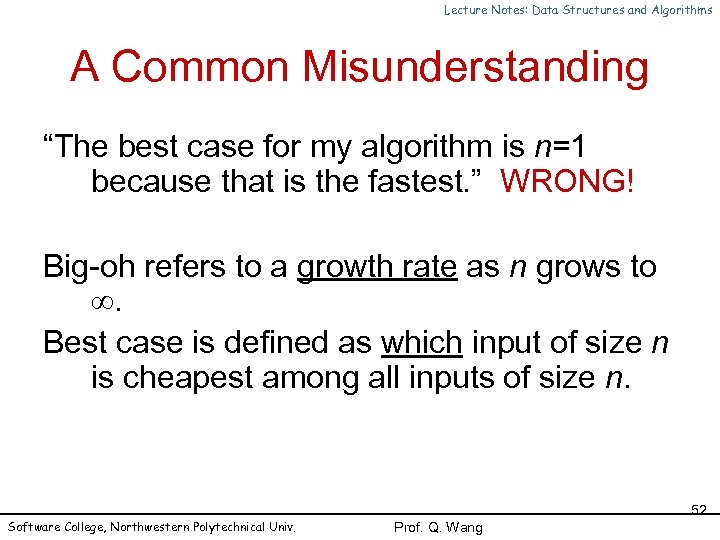Lecture Notes: Data Structures and Algorithms A Common Misunderstanding “The best case for my algorithm is n=1 because that is the fastest. ” WRONG! Big-oh refers to a growth rate as n grows to . Best case is defined as which input of size n is cheapest among all inputs of size n. Software College, Northwestern Polytechnical Univ. 52 Prof. Q. Wang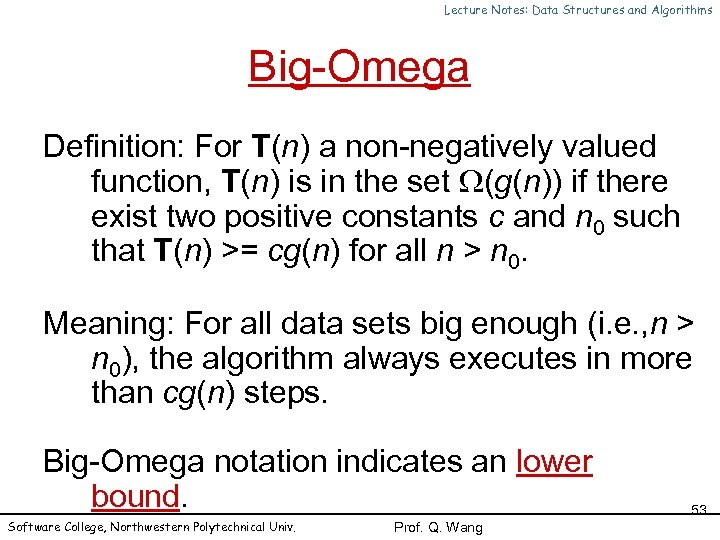Lecture Notes: Data Structures and Algorithms Big-Omega Definition: For T(n) a non-negatively valued function, T(n) is in the set (g(n)) if there exist two positive constants c and n 0 such that T(n) >= cg(n) for all n > n 0. Meaning: For all data sets big enough (i. e. , n > n 0), the algorithm always executes in more than cg(n) steps. Big-Omega notation indicates an lower bound. Software College, Northwestern Polytechnical Univ. Prof. Q. Wang 53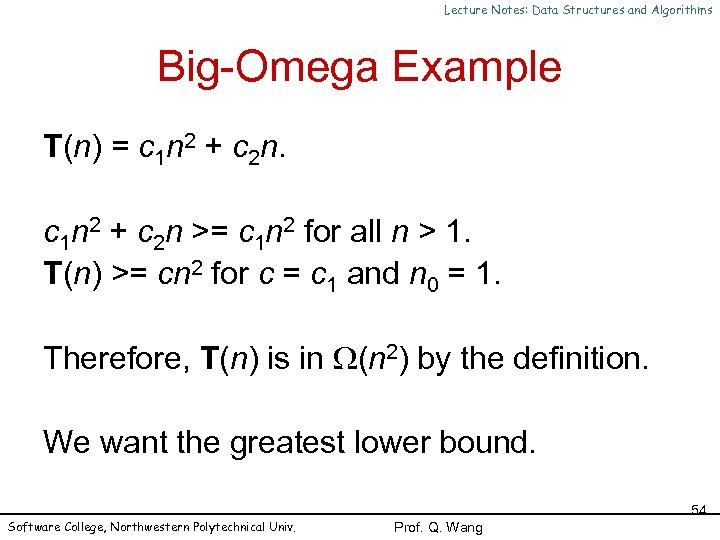Lecture Notes: Data Structures and Algorithms Big-Omega Example T(n) = c 1 n 2 + c 2 n >= c 1 n 2 for all n > 1. T(n) >= cn 2 for c = c 1 and n 0 = 1. Therefore, T(n) is in (n 2) by the definition. We want the greatest lower bound. Software College, Northwestern Polytechnical Univ. 54 Prof. Q. Wang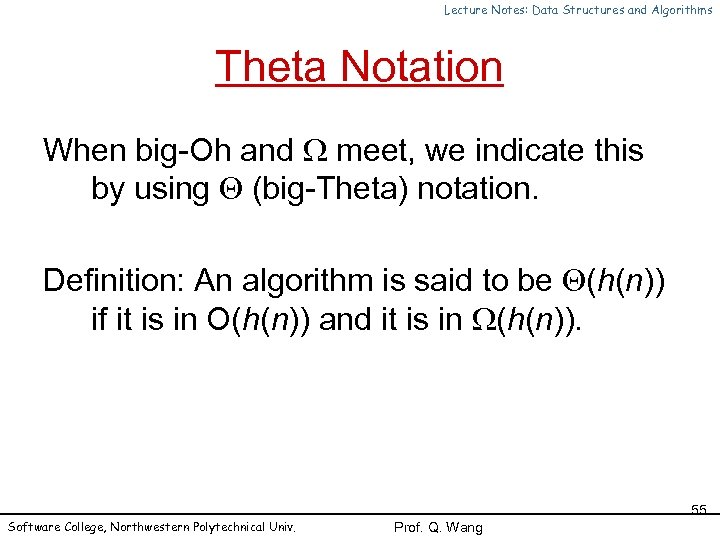Lecture Notes: Data Structures and Algorithms Theta Notation When big-Oh and meet, we indicate this by using (big-Theta) notation. Definition: An algorithm is said to be (h(n)) if it is in O(h(n)) and it is in (h(n)). Software College, Northwestern Polytechnical Univ. 55 Prof. Q. Wang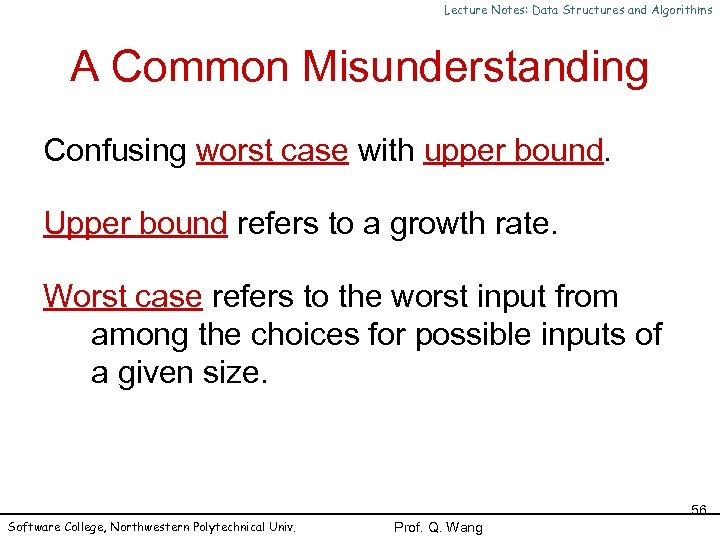Lecture Notes: Data Structures and Algorithms A Common Misunderstanding Confusing worst case with upper bound. Upper bound refers to a growth rate. Worst case refers to the worst input from among the choices for possible inputs of a given size. Software College, Northwestern Polytechnical Univ. 56 Prof. Q. Wang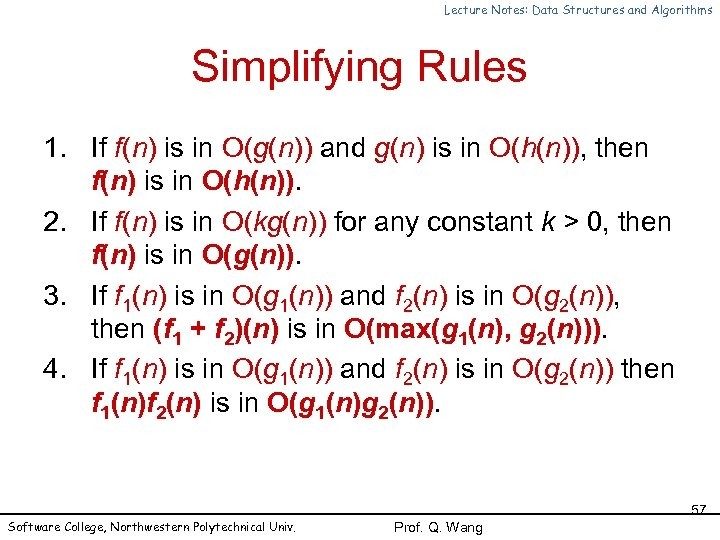Lecture Notes: Data Structures and Algorithms Simplifying Rules 1. If f(n) is in O(g(n)) and g(n) is in O(h(n)), then f(n) is in O(h(n)). 2. If f(n) is in O(kg(n)) for any constant k > 0, then f(n) is in O(g(n)). 3. If f 1(n) is in O(g 1(n)) and f 2(n) is in O(g 2(n)), then (f 1 + f 2)(n) is in O(max(g 1(n), g 2(n))). 4. If f 1(n) is in O(g 1(n)) and f 2(n) is in O(g 2(n)) then f 1(n)f 2(n) is in O(g 1(n)g 2(n)). Software College, Northwestern Polytechnical Univ. 57 Prof. Q. Wang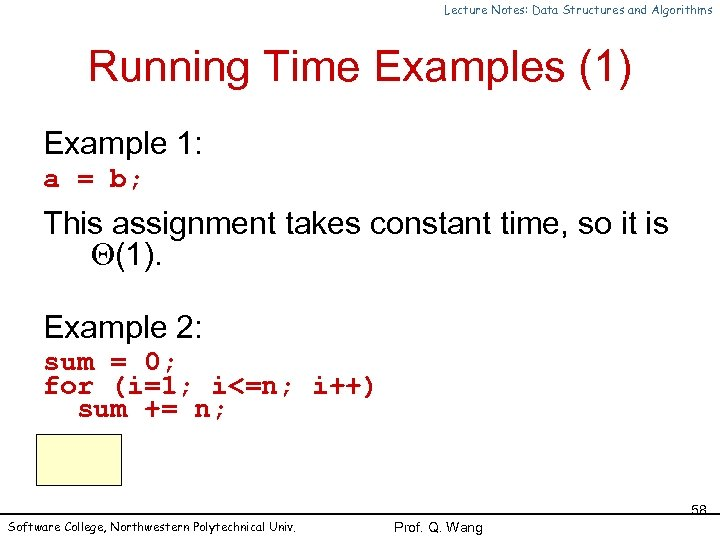Lecture Notes: Data Structures and Algorithms Running Time Examples (1) Example 1: a = b; This assignment takes constant time, so it is (1). Example 2: sum = 0; for (i=1; i<=n; i++) sum += n; O(n). Software College, Northwestern Polytechnical Univ. 58 Prof. Q. Wang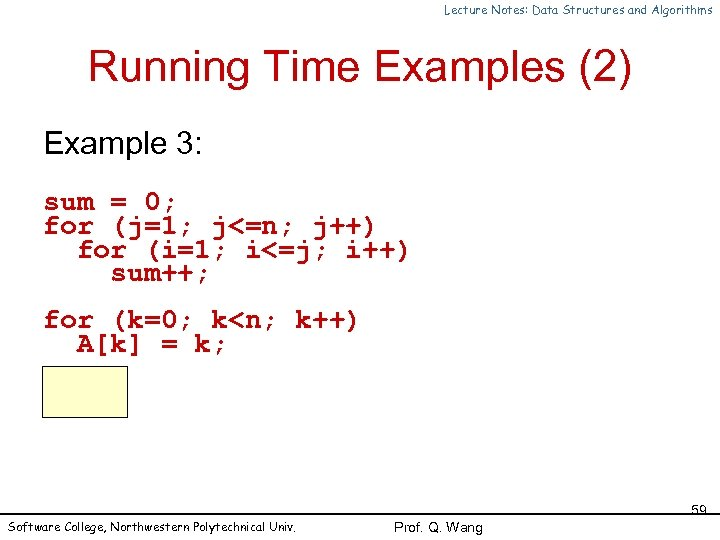Lecture Notes: Data Structures and Algorithms Running Time Examples (2) Example 3: sum = 0; for (j=1; j<=n; j++) for (i=1; i<=j; i++) sum++; for (k=0; k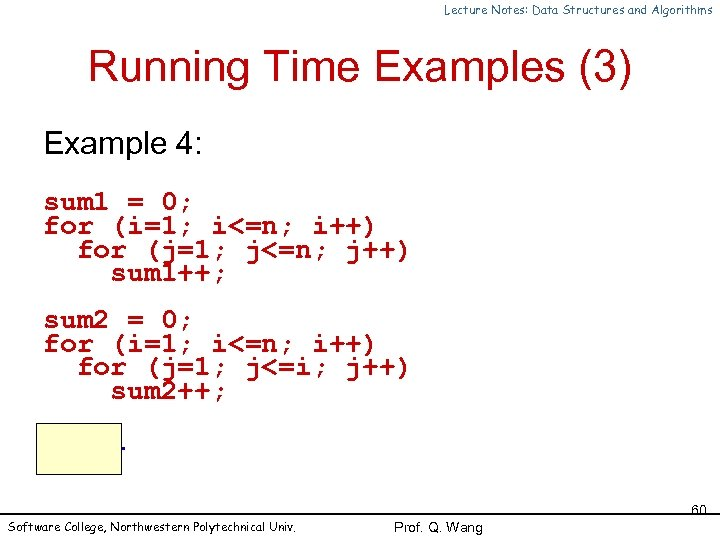Lecture Notes: Data Structures and Algorithms Running Time Examples (3) Example 4: sum 1 = 0; for (i=1; i<=n; i++) for (j=1; j<=n; j++) sum 1++; sum 2 = 0; for (i=1; i<=n; i++) for (j=1; j<=i; j++) sum 2++; O(n 2). Software College, Northwestern Polytechnical Univ. 60 Prof. Q. Wang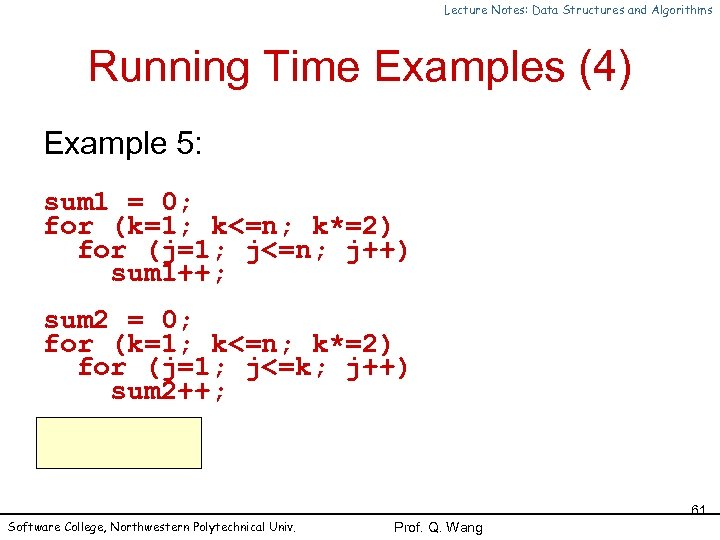Lecture Notes: Data Structures and Algorithms Running Time Examples (4) Example 5: sum 1 = 0; for (k=1; k<=n; k*=2) for (j=1; j<=n; j++) sum 1++; sum 2 = 0; for (k=1; k<=n; k*=2) for (j=1; j<=k; j++) sum 2++; O(nlog 2 n). Software College, Northwestern Polytechnical Univ. 61 Prof. Q. Wang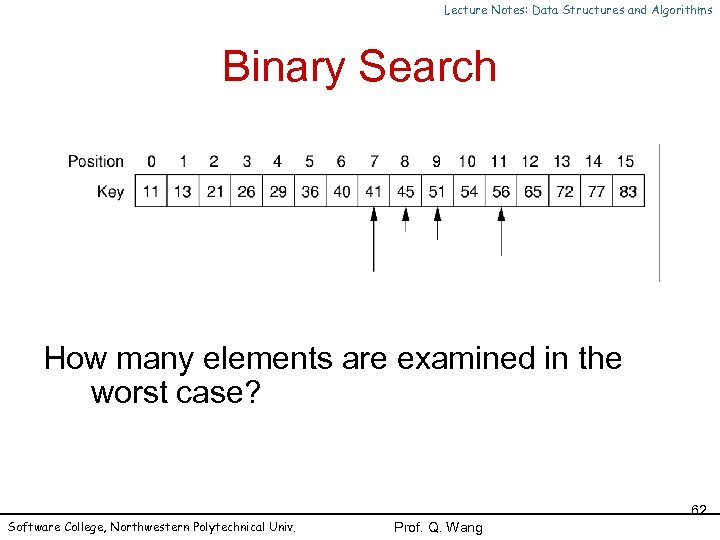Lecture Notes: Data Structures and Algorithms Binary Search How many elements are examined in the worst case? Software College, Northwestern Polytechnical Univ. 62 Prof. Q. Wang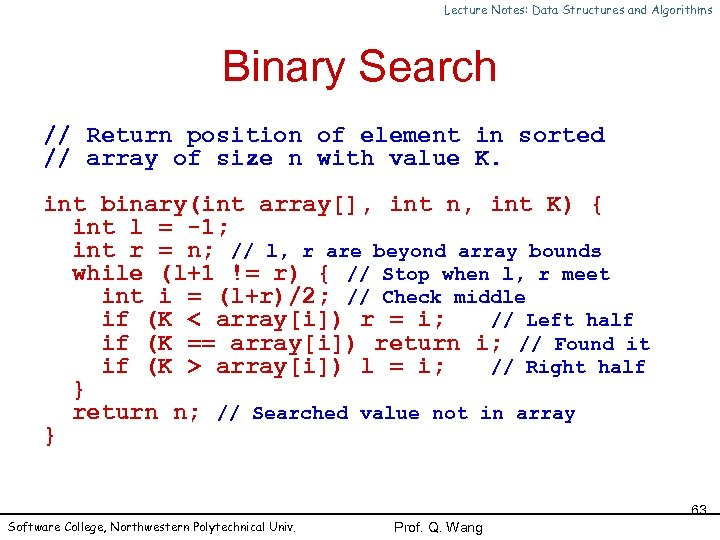Lecture Notes: Data Structures and Algorithms Binary Search // Return position of element in sorted // array of size n with value K. int binary(int array[], int n, int K) { int l = -1; int r = n; // l, r are beyond array bounds while (l+1 != r) { // Stop when l, r meet int i = (l+r)/2; // Check middle if (K < array[i]) r = i; // Left half if (K == array[i]) return i; // Found it if (K > array[i]) l = i; // Right half } return n; // Searched value not in array } Software College, Northwestern Polytechnical Univ. 63 Prof. Q. Wang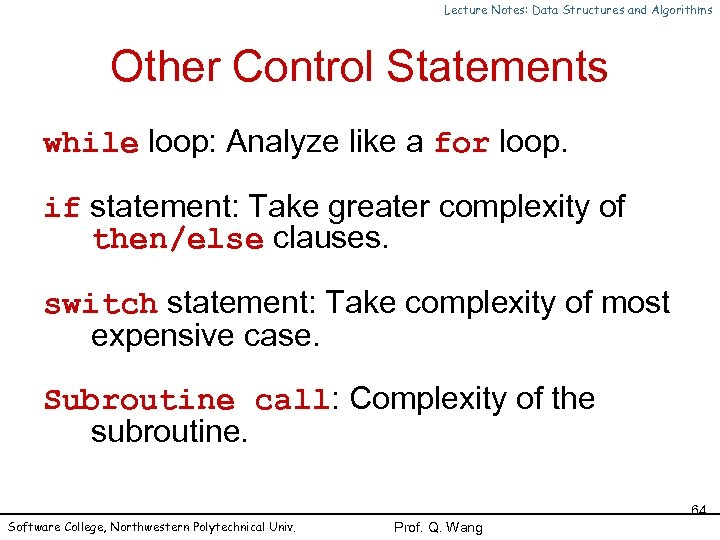Lecture Notes: Data Structures and Algorithms Other Control Statements while loop: Analyze like a for loop. if statement: Take greater complexity of then/else clauses. switch statement: Take complexity of most expensive case. Subroutine call: Complexity of the subroutine. Software College, Northwestern Polytechnical Univ. 64 Prof. Q. Wang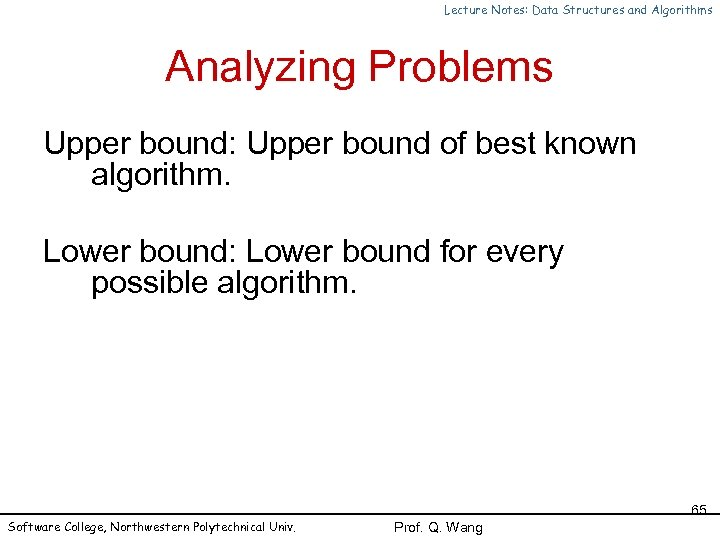Lecture Notes: Data Structures and Algorithms Analyzing Problems Upper bound: Upper bound of best known algorithm. Lower bound: Lower bound for every possible algorithm. Software College, Northwestern Polytechnical Univ. 65 Prof. Q. Wang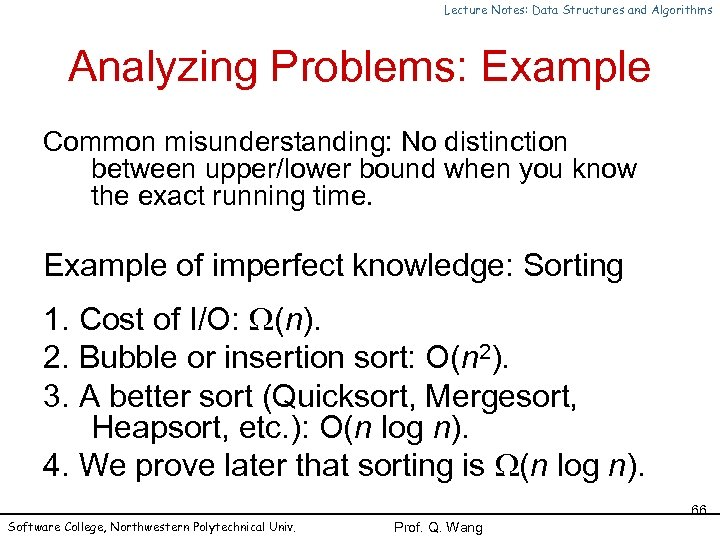Lecture Notes: Data Structures and Algorithms Analyzing Problems: Example Common misunderstanding: No distinction between upper/lower bound when you know the exact running time. Example of imperfect knowledge: Sorting 1. Cost of I/O: (n). 2. Bubble or insertion sort: O(n 2). 3. A better sort (Quicksort, Mergesort, Heapsort, etc. ): O(n log n). 4. We prove later that sorting is (n log n). Software College, Northwestern Polytechnical Univ. 66 Prof. Q. Wang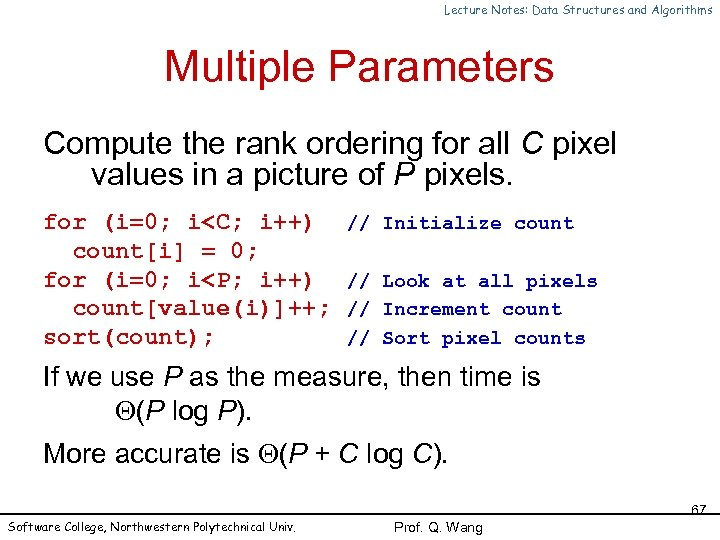Lecture Notes: Data Structures and Algorithms Multiple Parameters Compute the rank ordering for all C pixel values in a picture of P pixels. for (i=0; i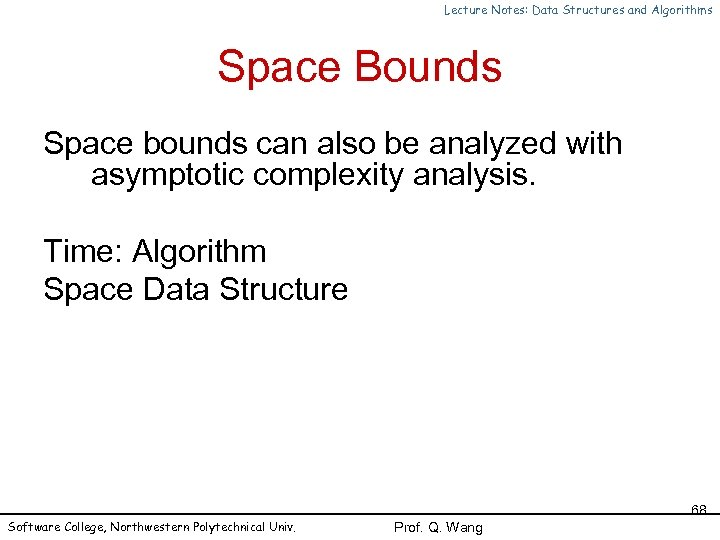Lecture Notes: Data Structures and Algorithms Space Bounds Space bounds can also be analyzed with asymptotic complexity analysis. Time: Algorithm Space Data Structure Software College, Northwestern Polytechnical Univ. 68 Prof. Q. Wang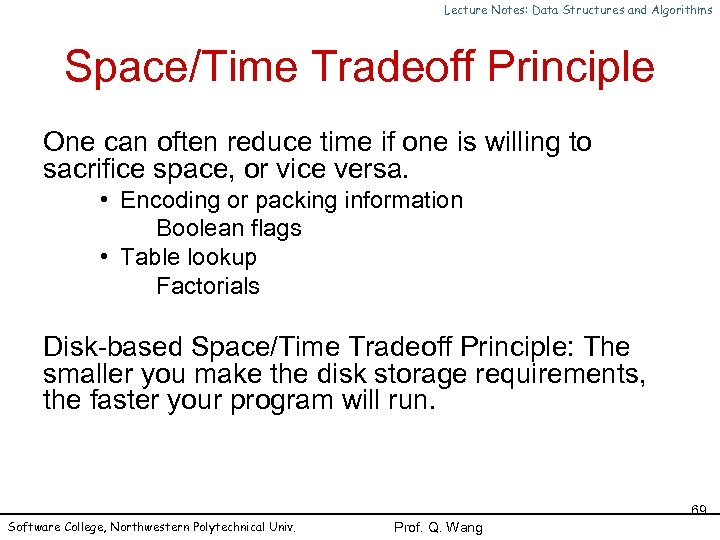Lecture Notes: Data Structures and Algorithms Space/Time Tradeoff Principle One can often reduce time if one is willing to sacrifice space, or vice versa. • Encoding or packing information Boolean flags • Table lookup Factorials Disk-based Space/Time Tradeoff Principle: The smaller you make the disk storage requirements, the faster your program will run. Software College, Northwestern Polytechnical Univ. 69 Prof. Q. Wang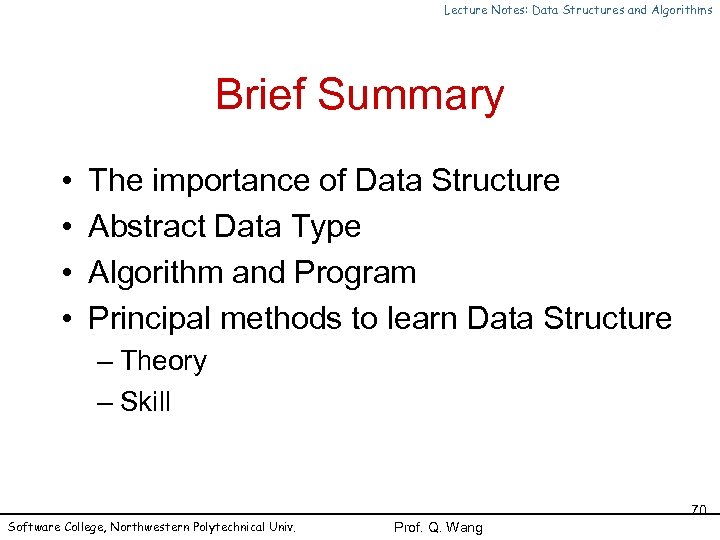Lecture Notes: Data Structures and Algorithms Brief Summary • • The importance of Data Structure Abstract Data Type Algorithm and Program Principal methods to learn Data Structure – Theory – Skill Software College, Northwestern Polytechnical Univ. 70 Prof. Q. Wang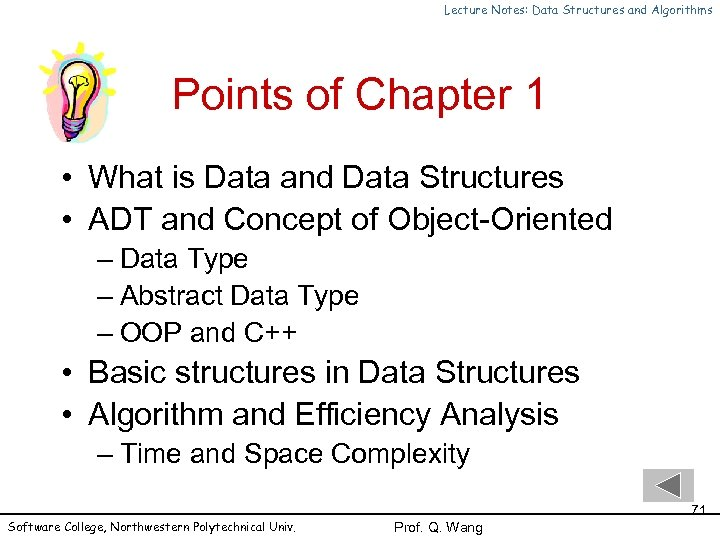Lecture Notes: Data Structures and Algorithms Points of Chapter 1 • What is Data and Data Structures • ADT and Concept of Object-Oriented – Data Type – Abstract Data Type – OOP and C++ • Basic structures in Data Structures • Algorithm and Efficiency Analysis – Time and Space Complexity Software College, Northwestern Polytechnical Univ. 71 Prof. Q. Wang